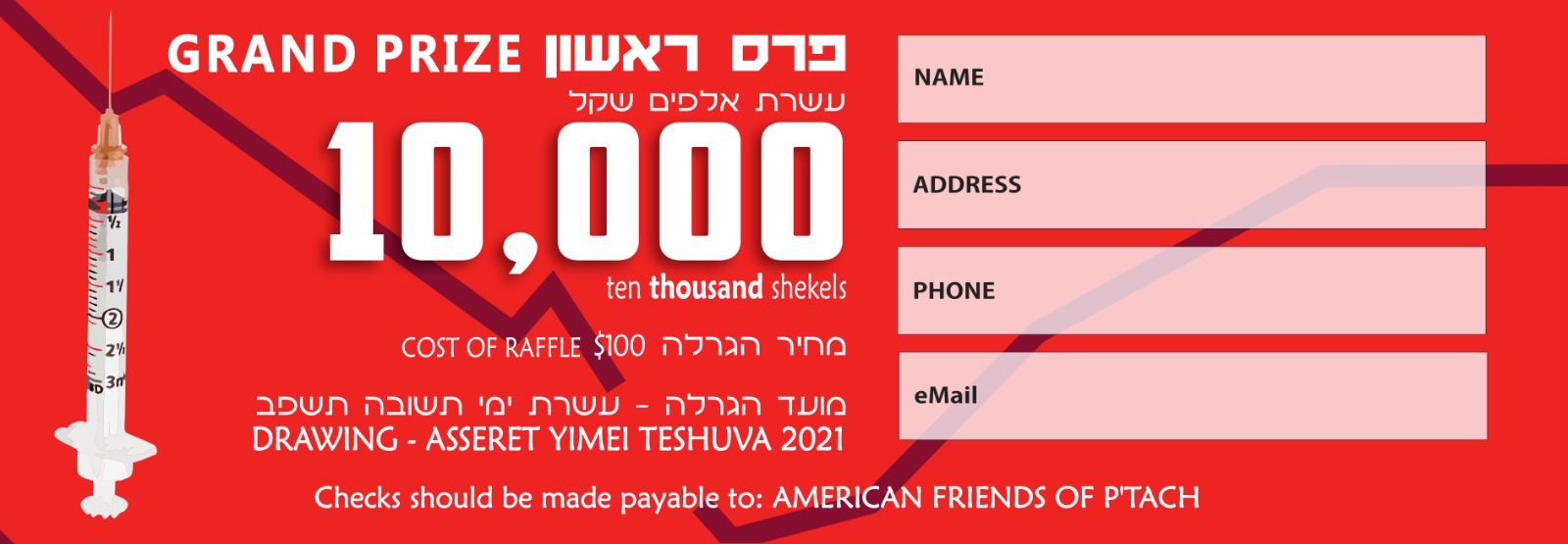## Scroll down for more info...• Shekel Bank Transfer

For shekel contributions you can make a bank transfer to:
BANK PAGI
BANK #52   BRANCH #182
PTACH-פתח לכל ילד
Account No. 690910This year in PTACH we helped over 1000 children overcome
their learning challenges and to succeed in school.

With your generous help, we can provide educational tools to a generation of children
- each with their own special needs and lay the groundwork for a successful future.

***************ABOUT
PTACH - Israel is a recognized 501c3 non-profit in the USA and a recognized charitable organization in Israel.
DONATE
Find us on Facebook
Send Us an eMail
\n", \$fdir); } // counter file in necessary dir: \$file = \$fdir . "/" . "counter.dat"; // already incremented a hit counter on this page, so passive mode in this one: if (isset(\$HitCounterFile) && (\$HitCounterFile == \$file)) { \$passive = true; } // create the file if it does not yet exist: if (!file_exists(\$file)) { file_put_contents(\$file,"",LOCK_EX); if (file_exists(\$file)) { chmod(\$file,0666); printf("\n", \$file); } else { printf("\n", \$file, getcwd()); } } else { if (!is_writable(\$file)) { chmod(\$file,0666); } if (\$passive) { printf("\n", \$file); } else { printf("\n", \$file); } } // get current count and add new entry: if (file_exists(\$file)) { if(isset(\$_SERVER['HTTP_X_FORWARDED_FOR']) && \$_SERVER['HTTP_X_FORWARDED_FOR'] != '') { \$yipaddr = \$_SERVER['HTTP_X_FORWARDED_FOR']; } else { \$yipaddr = \$_SERVER['REMOTE_ADDR']; } \$utime = time(); // check if ip address should be ignored: \$yignore = false; if ("" != "") { \$ipList = explode(" ", str_replace(array(",",";","\t","\n","\r"), " ", "")); if (in_array(\$yipaddr,\$ipList)) { \$yignore = true; } } else { \$ipList = array(); } // add a log entry if not passive mode, and even if ip address is ignored: if (!\$passive) { \$line = \$yipaddr . " " . \$utime . "\n"; file_put_contents(\$file,\$line,FILE_APPEND|LOCK_EX); } \$fline = file_get_contents(\$file); \$lines = explode("\n",\$fline); // get first time entry: \$firstTime = false; // sort on ip address: sort(\$lines); \$startCount = 5781; \$count = \$startCount; \$ips = \$startCount; \$timed = \$startCount; \$previp = ""; \$prevt = 0; foreach (\$lines as \$entry) { \$tokens = explode(" ",\$entry); if (count(\$tokens) == 2) { \$ipaddr = \$tokens; // filter ip addresses here: if (!in_array(\$ipaddr,\$ipList)) { \$utime = intval(\$tokens); if (\$firstTime === false) { \$firstTime = \$utime; } if (\$ipaddr != \$previp) \$ips ++; if ((\$ipaddr != \$previp) || ((\$utime - \$prevt) > 60)) \$timed ++; \$count ++; \$previp = \$ipaddr; \$prevt = \$utime; } } } // show count: \$usecount = \$count; // make a tip: \$details = \$count . " total hits, from " . \$ips . " unique IPs"; if (\$yignore) { \$details = \$details . " (not including yours " . \$yipaddr . ")"; } else { \$details = \$details . " (including yours " . \$yipaddr . ")"; } if (\$firstTime !== false) { date_default_timezone_set('UTC'); \$details = \$details . ", since " . date('l jS \of F Y h:i:s A',\$firstTime); } printf("", \$details); // split digits: \$str = "\$usecount"; \$strl = strlen(\$str); for (\$i=0; \$i<\$strl; \$i++) { if (\$i <= 0) { if (\$strl <= 1) { \$class = "xcounter"; } else { \$class = "fcounter"; } } else { if (\$i >= \$strl-1) { \$class = "lcounter"; } else { \$class = "counter"; } } if (("," != "") && (\$i > 0) && ((\$strl-\$i) % 3 == 0)) { printf(",", \$class); } printf("%s", \$class, substr(\$str,\$i,1)); } printf("\n"); // set this so another hit counter on this page will not increment again: if (!\$passive) { \$HitCounterFile = \$file; } } ?>